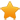Switch Editions?
CancelChannel: CodeSection,代码区,网络安全 - CodeSec
Viewing all articles

# Examining the Tweeting Patterns of Prominent Crossfit Gyms

0
0
A. Introduction

The growth of Crossfit has been one of the biggest developments in the fitnessindustry over the past decade. Promoted as both a physical exercise philosophy and also as a competitive fitness sport, Crossfit is a high-intensity fitness program incorporating elements from several sports and exercise protocols such as high-intensity interval training, Olympic weightlifting, plyometrics, powerlifting, gymnastics, strongman, and so forth. Now with over 10,000 Crossfit affiliated gyms (boxes) throughout the United States, the market has certainly become more saturated and gyms must initiate more unique marketing strategies to attract new members. In this post, I will investigate how some prominent Crossfit boxes are utilizing Twitter to engage with consumers. While Twitter is a great platform for news and entertainment, it is usually not the place for customer acquisition given the lack of targeted messaging. Furthermore, unlike platforms like Instagram,Twitter is simply not an image/video centric tool where followers can view accomplishments from their favorite fitness heroes, witness people achieving their goals, and so forth. Given these shortcomings, I wanted to understand how some prominent Crossfitboxes are actually using their Twitter accounts.

We begin by extracting the desired data from Twitter using the rtweet package in R. There are six prominent Crossfit gyms whose entire Twitter timeline we will use. To get this data, I looped through a vector containing each of their Twitter handles and used theget_timeline function to pull the desired data. Notice that there is a user defined function called add_engineered_features that is used to add a number of extra date columns. That function is available on my GitHub page here .

library(rtweet)
library(lubridate)
library(devtools)
library(data.table)
library(ggplot2)
library(hms)
library(scales)
# set working directory
setwd("~/Desktop/rtweet_crossfit")
final_dat.tmp <- list()
cf_gyms <- c("reebokcrossfit5", "crossfitmayhem", "crossfitsanitas", "sfcrossfit", "cfbelltown", "WindyCityCF")
for(each_box in cf_gyms){
message("Getting data for: ", each_box)
each_cf <- get_timeline(each_box, n = 3200) %>% data.table()
each_cf\$crossfit_name <- each_box
suppressWarnings( add_engineered_dates(each_cf, date_col = "created_at") )
final_dat.tmp[[each_box]] <- each_cf
message("")
}
final_dat <- rbindlist(final_dat.tmp)
final_dat\$contains_hashtags <- ifelse(!is.na(final_dat\$hashtags), 1, 0)
final_dat\$hashtags_count <- lapply(final_dat\$hashtags, function(x) length(x[!is.na(x)])) C. Exploratory Data Analysis

Let us start by investigating this data set to get a better understanding of trends and patterns across these various Crossfit boxes. The important thing to note is that notall these twitter accounts are currently active. We can see that crossfitmayhem, sfcrossfit, and WindyCityCF are the only ones who remain active.

final_dat[, .(min_max = range(as.Date(created_at))), by=crossfit_name][,label := rep(c("first_tweet","last_tweet"))][] C1. Total Number of Tweets

Sfcrossfit, which is the oldest of these gyms, has the highest number of tweets. However, when looking at the total number of tweets per active month, they were less active than two other gyms.

## total number of tweets
p1 = final_dat[, .N, by=crossfit_name] %&amp;gt;%
ggplot(., aes(x=reorder(crossfit_name, N), y=N)) + geom_bar(stat='identity', fill="steelblue") +
coord_flip() + labs(x="", y="") + ylim(0,3000) + ggtitle("Total Number of Tweets") +
theme(plot.title = element_text(hjust = 0.5),
axis.ticks.x = element_line(colour = "black"),
axis.ticks.y = element_line(colour = "black"))
## number of tweets per active month
p2 = final_dat[, .(.N, start=lubridate::ymd_hms(min(created_at)), months_active=lubridate::interval(lubridate::ymd_hms(min(created_at)), Sys.Date()) %/% months(1)), by=crossfit_name][,
.(tweets_per_month = N/months_active), by=crossfit_name] %&amp;gt;%
ggplot(., aes(x=reorder(crossfit_name, tweets_per_month), y=tweets_per_month)) +
geom_bar(stat='identity', fill="steelblue") + coord_flip() + labs(x="", y="") + ylim(0,32) +
theme(plot.title = element_text(hjust = 0.5),
axis.ticks.x = element_line(colour = "black"),
axis.ticks.y = element_line(colour = "black")) +
ggtitle("Total Number of Tweets per Active Month")
## add both plots to a single pane
grid.arrange(p1, p2, nrow=1)

C2. Total Number of Tweets Over Time

The time series for the total number of tweets by month shows that each gym had one or two peaks from 2012 through 2016 where they were aggressively sharing content with their followers. However, over the past two years, each gym has reduced their twitter usage significantly.

## total number of tweets by month
final_dat[, .N, by = .(crossfit_name, created_at_YearMonth)][order(crossfit_name, created_at_YearMonth)][,
created_at_YearMonth := lubridate::ymd(paste(created_at_YearMonth, "-01"))] %&amp;gt;%
ggplot(., aes(created_at_YearMonth, N, colour=crossfit_name)) + geom_line(group=1, lwd=0.6) +
facet_wrap(~crossfit_name) + labs(x="", y="") + theme(legend.position="none") +
theme(plot.title = element_text(hjust = 0.5),
axis.ticks.x = element_line(colour = "black"),
axis.ticks.y = element_line(colour = "black"),
strip.text.x = element_text(size = 10)) +
ggtitle("Total Number of Tweets")
## total number of tweets by year
ggplot(data = final_dat,
aes(lubridate::month(created_at, label=TRUE, abbr=TRUE),
group=factor(lubridate::year(created_at)), color=factor(lubridate::year(created_at))))+
geom_line(stat="count") + geom_point(stat="count") +
facet_wrap(~crossfit_name) + labs(x="", colour="Year") + xlab("") + ylab("") +
theme(plot.title = element_text(hjust = 0.5),
axis.ticks.x = element_line(colour = "black"),
axis.ticks.y = element_line(colour = "black"),
strip.text.x = element_text(size = 10)) +
ggtitle("Total Number of Tweets by Year")

C3. Tweeting Volume by Year, Month, and Day

For each Crossfit gym, I plotted the volume of tweets by year, month, and day. Oddly enough, there really are not any noticeable patterns in these charts.

## years with the highest number of tweets
ggplot(final_dat, aes(created_at_Year)) + geom_bar(fill="steelblue") +
facet_wrap(~crossfit_name) + labs(x="", y="") +
theme(plot.title = element_text(hjust = 0.5),
axis.ticks.x = element_line(colour = "black"),
axis.ticks.y = element_line(colour = "black"),
strip.text.x = element_text(size = 10)) + ylim(0,800) +
ggtitle("Total Number of Tweets by Year")
## months with the highest number of tweets
final_dat[, created_at_YearMonth2 := lubridate::ymd(paste(created_at_YearMonth, "-01"))][] %&amp;gt;%
ggplot(., aes(lubridate::month(created_at_YearMonth2, label=TRUE, abbr=TRUE))) + geom_bar(fill="steelblue") +
facet_wrap(~crossfit_name) + labs(x="", y="") +
theme(plot.title = element_text(hjust = 0.5),
axis.ticks.x = element_line(colour = "black"),
axis.ticks.y = element_line(colour = "black"),
strip.text.x = element_

Viewing all articles

### 出售: CV378 5Z3 5U4G U52 GZ34 U50 5Y3 EZ150 5V4

More Pages to Explore .....